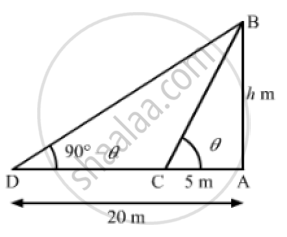# The Angles of Elevation of the Top of a Tower from Two Points at Distance of 5 Metres and 20 Metres from the Base of the Tower and is the Same Straight Line with It, . Find the Height of the Tower. - Mathematics

The angles of elevation of the top of a tower from two points at distance of 5 metres and 20 metres from the base of the tower and is the same straight line with it, are complementary. Find the height of the tower.

#### SolutionLet the height of the tower be AB.
We have.
AC = 5m, AD = 20m
Let the angle of elevation of the top of the tower (i.e. ∠ACB) from point C be θ .
Then,
the angle of elevation of the top of the tower (i.e. Z ADB) from point D
=(90° -θ)
Now, in ΔABC

tan theta = (AB)/(AC)

⇒ tan theta = (AB)/5            ...................(i)

Also, in ΔABD,

cot (90° - theta ) = (AD)/(AB)

⇒  tan theta = 20/(AB)                .................(ii)

From (i) and (ii), we get

(AB)/5 = 20/(AB)

⇒ AB^2 = 100

⇒  AB = sqrt(100)

∴ AB = 10 m

So, the height of the tower is 10 m.

Concept: Heights and Distances
Is there an error in this question or solution?
Chapter 14: Height and Distance - Exercises

#### APPEARS IN

RS Aggarwal Secondary School Class 10 Maths
Chapter 14 Height and Distance
Exercises | Q 4

Share